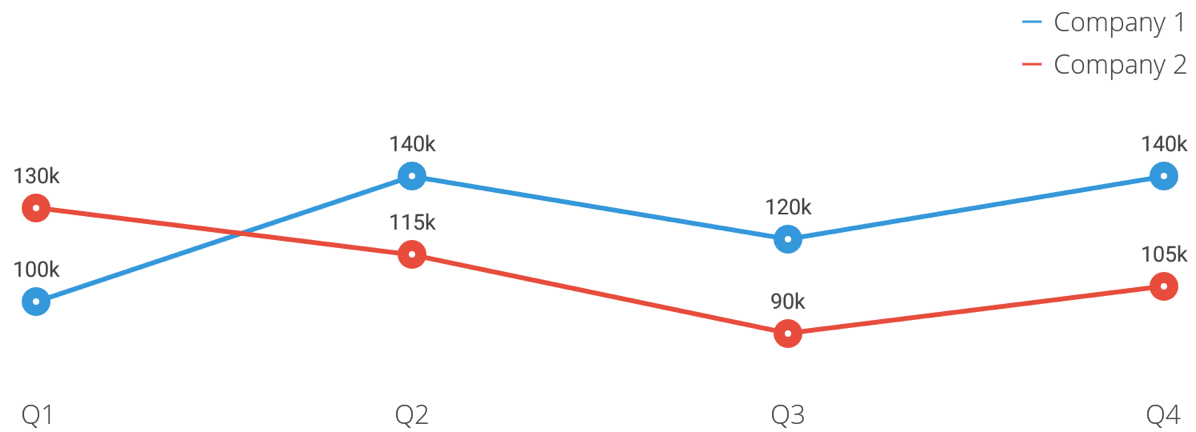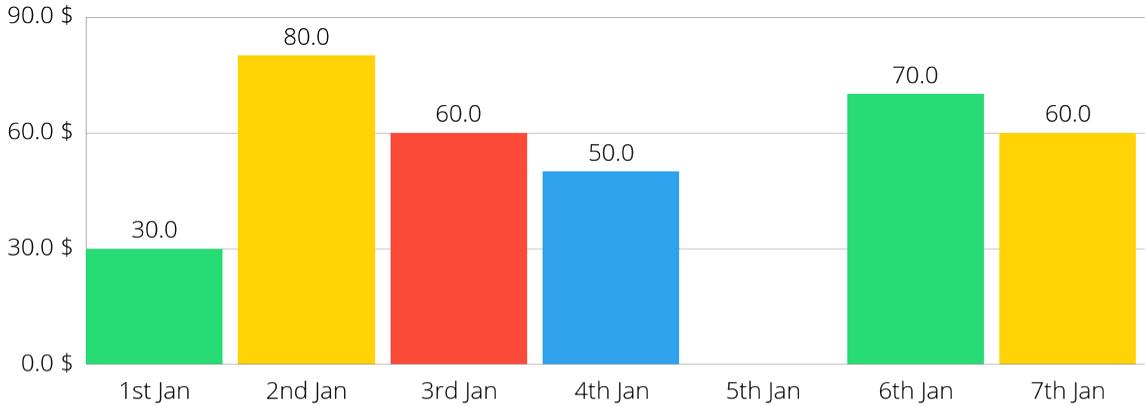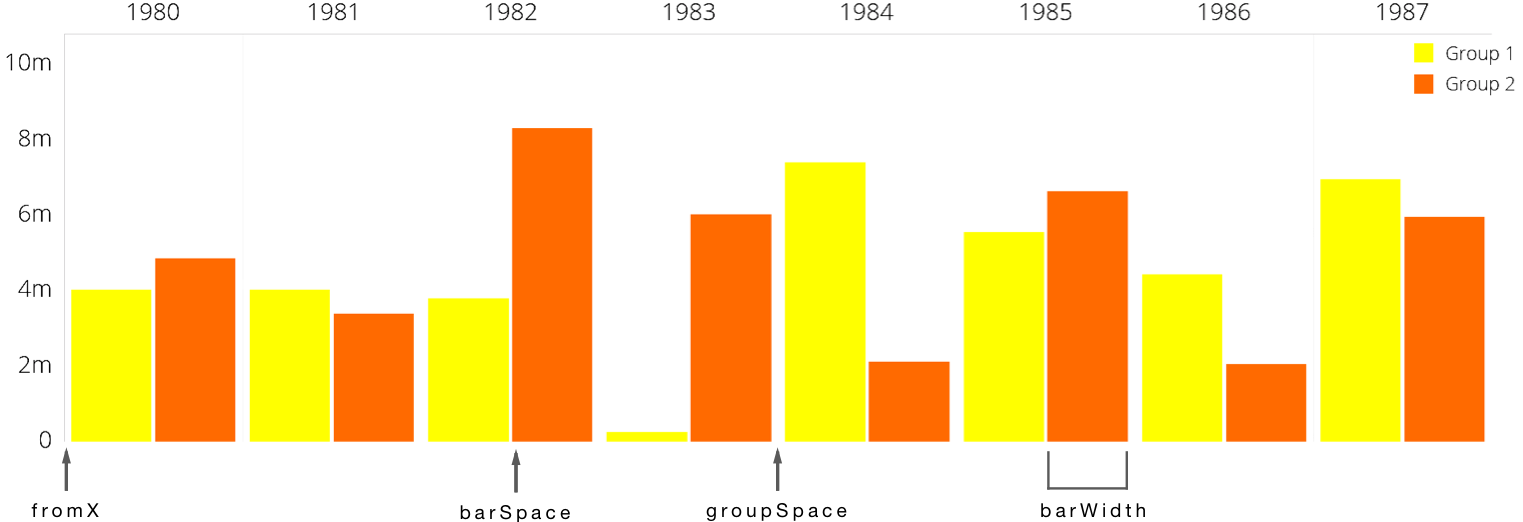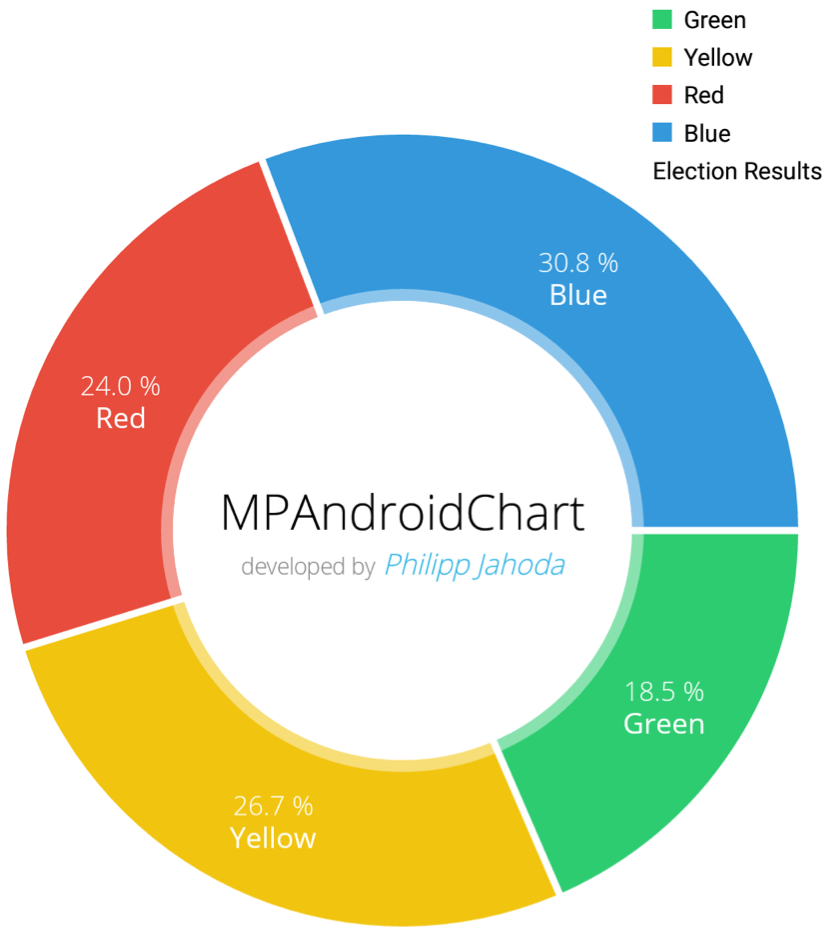MPAndroidChart 教程：设置数据 Setting Data

0
0
01. 云栖社区>
2. 博客>
3. 正文

MPAndroidChart 教程：设置数据 Setting Data

线型图

public void setData(ChartData data) { ... }

/** List constructor */
public LineData(List<ILineDataSet> sets) { ... }

/** Constructor with one or multiple ILineDataSet objects */
public LineData(ILineDataSet...) { ... }

public LineDataSet(List<Entry> entries, String label) { ... }

Entry类型列表封装了图表的所有值。Entry对象是图表中具有x值和y值的条目的附加包装器：

public Entry(float x, float y) { ... }

List<Entry> valsComp1 = new ArrayList<Entry>();
List<Entry> valsComp2 = new ArrayList<Entry>();

Entry c1e1 = new Entry(0f, 100000f); // 0 == quarter 1
Entry c1e2 = new Entry(1f, 140000f); // 1 == quarter 2 ...
// and so on ...

Entry c2e1 = new Entry(0f, 130000f); // 0 == quarter 1
Entry c2e2 = new Entry(1f, 115000f); // 1 == quarter 2 ...
//...

LineDataSet setComp1 = new LineDataSet(valsComp1, "Company 1");
setComp1.setAxisDependency(AxisDependency.LEFT);
LineDataSet setComp2 = new LineDataSet(valsComp2, "Company 2");
setComp2.setAxisDependency(AxisDependency.LEFT);

// use the interface ILineDataSet
List<ILineDataSet> dataSets = new ArrayList<ILineDataSet>();

LineData data = new LineData(dataSets);
mLineChart.setData(data);
mLineChart.invalidate(); // refresh

// the labels that should be drawn on the XAxis
final String[] quarters = new String[] { "Q1", "Q2", "Q3", "Q4" };

IAxisValueFormatter formatter = new IAxisValueFormatter() {

@Override
public String getFormattedValue(float value, AxisBase axis) {
return quarters[(int) value];
}

// we don't draw numbers, so no decimal digits needed
@Override
public int getDecimalDigits() {  return 0; }
};

XAxis xAxis = mLineChart.getXAxis();
xAxis.setGranularity(1f); // minimum axis-step (interval) is 1
xAxis.setValueFormatter(formatter);条目的顺序

List<Entry> entries = ...; Collections.sort(entries, new EntryXComparator());

BarChart

List<BarEntry> entries = new ArrayList<>();
// gap of 2f

BarDataSet set = new BarDataSet(entries, "BarDataSet");

BarData data = new BarData(set);
data.setBarWidth(0.9f); // set custom bar width
chart.setData(data);
chart.setFitBars(true); // make the x-axis fit exactly all bars
chart.invalidate(); // refreshGrouped BarChart

YourData[] group1 = ...;
YourData[] group2 = ...;

List<BarEntry> entriesGroup1 = new ArrayList<>();
List<BarEntry> entriesGroup2 = new ArrayList<>();

// fill the lists
for(int i = 0; i < group1.length; i++) {
}

BarDataSet set1 = new BarDataSet(entriesGroup1, "Group 1");
BarDataSet set2 = new BarDataSet(entriesGroup2, "Group 2");

float groupSpace = 0.06f;
float barSpace = 0.02f; // x2 dataset
float barWidth = 0.45f; // x2 dataset
// (0.02 + 0.45) * 2 + 0.06 = 1.00 -> interval per "group"

BarData data = new BarData(set1, set2);
data.setBarWidth(barWidth); // set the width of each bar
barChart.setData(data);
barChart.groupBars(1980f, groupSpace, barSpace); // perform the "explicit" grouping
barChart.invalidate(); // refresh

public void groupBars(float fromX, float groupSpace, float barSpace) { ... }

fromX参数确定XAxis分组条的起始位置（在本例中为“1980”），groupSpace确定每组条之间留下的空间，barSpace确定组中各个条之间的间距。基于这些参数，该groupBars(...)方法改变每个条形XAxis朝向分组外观的位置，保持各个BarEntry对象的顺序。XAxis xAxis = chart.getXAxis();
xAxis.setCenterAxisLabels(true);

Stacked BarChart

public BarEntry(float x, float [] yValues) { ... }

BarEntry stackedEntry = new BarEntry(0f, new float[] { 10, 20, 30 });

BarEntry由三个值组成，具有“高度”为“10”，“20”和“30”。

PieChart

public PieEntry(float value, String label) { ... }

List<PieEntry> entries = new ArrayList<>();

PieDataSet set = new PieDataSet(entries, "Election Results");
PieData data = new PieData(set);
pieChart.setData(data);
pieChart.invalidate(); // refresh

PieEntry对象不保存x位置的值，因为图表中显示的PieEntry对象的顺序由它们在条目列表中的顺序确定。+ 关注